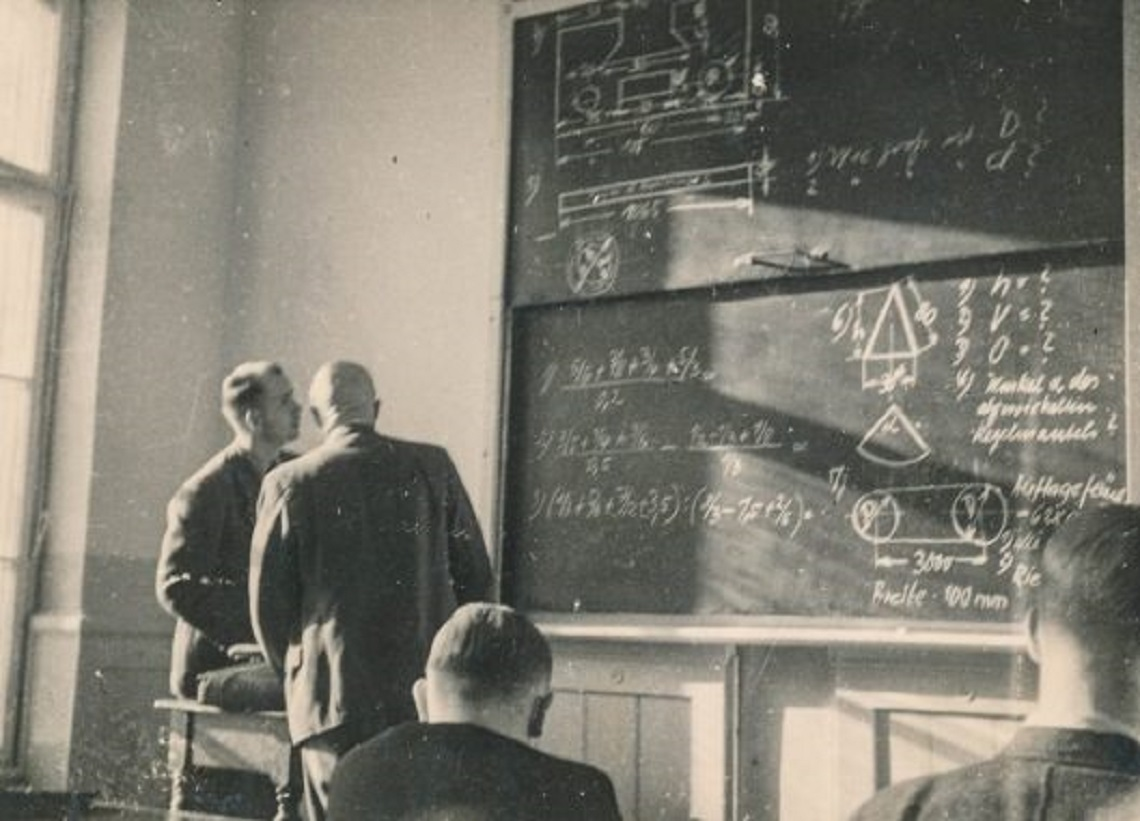## Auctioneers, Alpha and Beta and more …

#### By

Mike Brandly
1 April 2019 - 4:14pm

In 2010, we modeled a formula for finding the odds of the most interested bidder (Alpha) and the second most interested bidder (Beta) attending an auction. We searched for that formula since all auctioneers really need are the highest bidder and the second highest bidder.

Of course, the issue is — who is the most interested bidder? Who is the second most interested bidder? Since prior to the auction, these people are largely unknown to the auctioneer/seller, it is prudent to maximize the number of bidders in order to maximize the chances of having the most interested bidder and second most interested bidder.

That prior treatise and associated details can be found here: https://mikebrandlyauctioneer.wordpress.com/2010/01/12/auctioneer-looking-for-alpha-and-beta/.

Relatedly, there is the issue of a large crowd over a small crowd even if there are only two bidders. We discussed this phenominom here: https://mikebrandlyauctioneer.wordpress.com/2016/05/31/large-auction-crowds-busy-restaurants-and-relevance/.

Today, we expand on our prior formula, noting the odds of not having Alpha nor Beta, Alpha and not Beta, and Beta and not Alpha. First, here is the formula for the odds of having both Alpha and Beta (where B ≥ 0):

B = number of bidders

X = chances of having Alpha and Beta present
X = [[[(2*B)/(B+1)]-1]*min{B,1}]^2

Here are our additional formulas (where B ≥ 2):

Y = chances of not having Alpha and Beta present
Y = 1-[[[[(2*B)/(B+1)]-1]*min{B,1}]^2] or 1-X

Y1 = chances of having Alpha but not Beta present
Y1 = [1-[[[[(2*B)/(B+1)]-1]*min{B,1}]^2]]/2 or Y/2

Y2 = chances of having Beta but not Alpha present
Y2 = [[[(2*B)/(B+1)]-1]*min{B,1}]^2/[[[[(2*B)/(B+1)]-1]*min{B,1}]^2]+1-[[[[(2*B)/(B+1)]-1]*min{B,1}]^2]]/2]-[[[(2*B)/(B+1)]-1]*min{B,1}]^2 or X/[X+Y1]-X

Y3 = chances of not having Alpha nor Beta present
Y3 = [1-[[[[(2*B)/(B+1)]-1]*min{B,1}]^2]]/2 – [[[[(2*B)/(B+1)]-1]*min{B,1}]^2]^2 or Y/2-Y2

Therefore, we have these two additional equations:

Y = Y1 + Y2 + Y3

X = (X + Y1)*min{X,1} * (X + Y2)*min{X,1}
_________________________________________

Here’s an example:
If an auctioneer is selling one item, and has 6 bidders:

There is a 51.02% chance (X) Alpha and Beta will be present.
There is a 48.98% chance (Y) Alpha and Beta will not be present.
There is a 24.49% chance (Y1) Alpha will be present without Beta.
There is a 16.55% chance (Y2) Beta will be present without Alpha.
There is a 7.94% (Y3) neither Alpha nor Beta will be present.

Alpha and Beta are both not there (Y) 48.98% of the time.
0.2449 (Y1) + 0.1655 (Y2) + 0.0794 (Y3) is Y or 48.98%

Alpha is there (X + Y1) 75.51% of the time.
Beta is there (X + Y2) 67.57% of the time.
0.7551*min{X,1} * 0.6757*min{X,1} is X or 51.02%
_________________________________________

The two special cases (not necessarily material) outside of the purview of these last formulas where B = 0 or B = 1 require more mathematical observation:

B = 0, X = 0%, Y = 100%, Y1 = 0%, Y2 = 0%, Y3 = 100%
Alpha is there (X + Y1) 0% of the time.
Beta is there (X + Y2) 0% of the time.
0*min{X,1} * 0*min{X,1} is X or 0%

B = 1, X = 0%, Y = 100%, Y1 = 22.22%, Y2 = 4.44%, Y3 = 73.33%
Alpha is there (X + Y1) 22.22% of the time.
Beta is there (X + Y2) 4.44% of the time.
0.2222*min{X,1} * 0.0444*min{X,1} is X or 0%
_________________________________________

And for comparative purposes, here is the same for B = 2, 3 and 4:

B = 2, X = 11.11%, Y = 88.89%, Y1 = 44.45%, Y2 = 8.89%, Y3 = 35.56%
Alpha is there (X + Y1)*min{X,1} 55.56% of the time.
Beta is there (X + Y2) 20.00% of the time.
0.55*min{X,1} * 0.20*min{X,1} is X or 11.11%

B = 3, X = 25.00%, Y = 75.00%, Y1 = 37.50%, Y2 = 15.00%, Y3 = 22.50%
Alpha is there (X + Y1)*min{X,1} 62.50% of the time.
Beta is there (X + Y2) 40.00% of the time.
0.6250*min{X,1} * 0.40*min{X,1} is X or 25.00%

B = 4, X = 36.00%, Y = 64.00%, Y1 = 32.00%, Y2 = 16.94%, Y3 = 15.06%
Alpha is there (X + Y1)*min{X,1} 68.00% of the time.
Beta is there (X + Y2) 52.94% of the time.
0.68*min{X,1} * 0.5294*min{X,1} is X or 36.00%

As should be noted, the chances of having Alpha and Beta at an auction (X) increase with the number of bidders (B,) but increase at an increasingly slower pace (0%, 11%, 25%, 36%, 44%, 51%, 56%, 60%, 64%, 67%, 69%, 72%, 73%, 75%, 77%, 78%, 79% …)

A good lesson for auctioneers is that there is a point that the advantages of one more bidder is outweighed by the expense of securing such bidder.